# 10.5 Graphing quadratic equations  (Page 5/15)

 Page 5 / 15

Finding the y -intercept by substituting $x=0$ into the equation is easy, isn’t it? But we needed to use the Quadratic Formula to find the x -intercepts in [link] . We will use the Quadratic Formula again in the next example.

Graph $y=2{x}^{2}-4x-3$ .

## Solution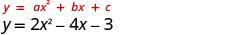The equation y has one side. Since a is 2, the parabola opens upward.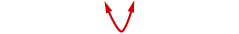To find the axis of symmetry, find $x=-\frac{b}{2a}$ .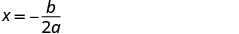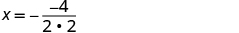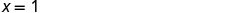The axis of symmetry is $x=1$ . The vertex on the line $x=1.$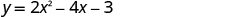Find y when $x=1$ .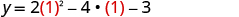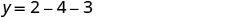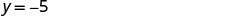The vertex is $\left(1,\text{−}5\right)$ . The y -intercept occurs when $x=0.$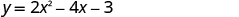Substitute $x=0.$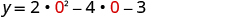Simplify.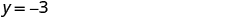The y- intercept is $\left(0,-3\right)$ . The point $\left(0,-3\right)$ is one unit to the left of the line of symmetry. The point one unit to the right of the line of symmetry is $\left(2,-3\right)$ Point symmetric to the y- intercept is $\left(2,-3\right).$ The x -intercept occurs when $y=0$ .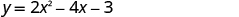Substitute $y=0$ .Use the Quadratic Formula.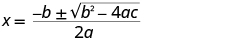Substitute in the values of a, b, c.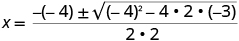Simplify.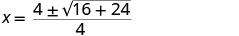Simplify inside the radical.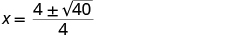Simplify the radical.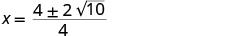Factor the GCF.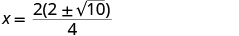Remove common factors.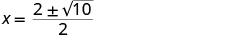Write as two equations.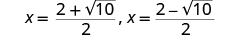Approximate the values.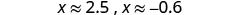The approximate values of the x- intercepts are $\left(2.5,0\right)$ and $\left(-0.6,0\right)$ . Graph the parabola using the points found.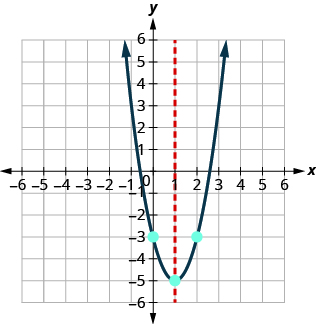Graph the parabola $y=5{x}^{2}+10x+3.$

$y\text{:}\phantom{\rule{0.2em}{0ex}}\left(0,3\right);\phantom{\rule{0.2em}{0ex}}x\text{:}\phantom{\rule{0.2em}{0ex}}\left(-1.6,0\right),\left(-0.4,0\right);$
axis: $x=-1;\phantom{\rule{0.2em}{0ex}}\text{vertex:}\phantom{\rule{0.2em}{0ex}}\left(-1,-2\right)$ ;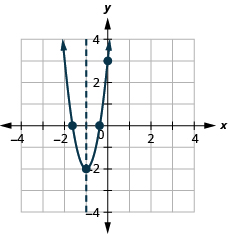Graph the parabola $y=-3{x}^{2}-6x+5.$

$y\text{:}\phantom{\rule{0.2em}{0ex}}\left(0,5\right);\phantom{\rule{0.2em}{0ex}}x\text{:}\phantom{\rule{0.2em}{0ex}}\left(0.6,0\right),\left(-2.6,0\right);$
axis: $x=-1;\phantom{\rule{0.2em}{0ex}}\text{vertex:}\phantom{\rule{0.2em}{0ex}}\left(-1,8\right)$ ;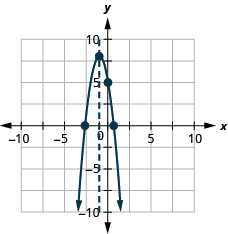## Solve maximum and minimum applications

Knowing that the vertex    of a parabola is the lowest or highest point of the parabola gives us an easy way to determine the minimum or maximum value of a quadratic equation. The y -coordinate of the vertex is the minimum y -value of a parabola that opens upward. It is the maximum y -value of a parabola that opens downward. See [link] .

## Minimum or maximum values of a quadratic equation

The y -coordinate of the vertex of the graph of a quadratic equation is the

• minimum value of the quadratic equation if the parabola opens upward.
• maximum value of the quadratic equation if the parabola opens downward.

Find the minimum value of the quadratic equation $y={x}^{2}+2x-8$ .

## Solution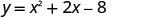Since a is positive, the parabola opens upward. The quadratic equation has a minimum. Find the axis of symmetry.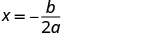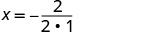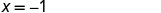The axis of symmetry is $x=-1$ . The vertex is on the line $x=-1.$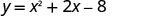Find y when $x=-1.$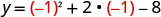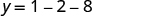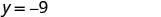The vertex is $\left(-1,-9\right)$ . Since the parabola has a minimum, the y- coordinate of the vertex is the minimum y- value of the quadratic equation. The minimum value of the quadratic is $-9$ and it occurs when $x=-1$ . Show the graph to verify the result.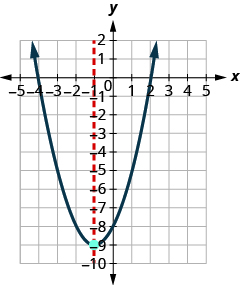Find the maximum or minimum value of the quadratic equation $y={x}^{2}-8x+12$ .

The minimum value is $-4$ when $x=4$ .

Find the maximum or minimum value of the quadratic equation $y=-4{x}^{2}+16x-11$ .

The maximum value is 5 when $x=2$ .

We have used the formula

$h=-16{t}^{2}+{v}_{0}t+{h}_{0}$

to calculate the height in feet, $h$ , of an object shot upwards into the air with initial velocity, ${v}_{0}$ , after $t$ seconds.

This formula is a quadratic equation in the variable $t$ , so its graph is a parabola. By solving for the coordinates of the vertex, we can find how long it will take the object to reach its maximum height. Then, we can calculate the maximum height.

The quadratic equation $h=-16{t}^{2}+{v}_{0}t+{h}_{0}$ models the height of a volleyball hit straight upwards with velocity 176 feet per second from a height of 4 feet.

1. How many seconds will it take the volleyball to reach its maximum height?
2. Find the maximum height of the volleyball.

## Solution

$h=-16{t}^{2}+176t+4$

Since a is negative, the parabola opens downward.

The quadratic equation has a maximum.

1. $\begin{array}{cccc}\text{Find the axis of symmetry.}\hfill & & & \phantom{\rule{4em}{0ex}}\begin{array}{c}t=-\frac{b}{2a}\hfill \\ t=-\frac{176}{2\left(-16\right)}\hfill \\ t=5.5\hfill \end{array}\hfill \\ & & & \phantom{\rule{4em}{0ex}}\text{The axis of symmetry is}\phantom{\rule{0.2em}{0ex}}t=5.5.\hfill \\ \text{The vertex is on the line}\phantom{\rule{0.2em}{0ex}}t=5.5.\hfill & & & \phantom{\rule{4em}{0ex}}\text{The maximum occurs when}\phantom{\rule{0.2em}{0ex}}t=5.5\phantom{\rule{0.2em}{0ex}}\text{seconds.}\hfill \end{array}$

2.  Find h when $t=5.5$ .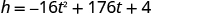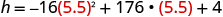Use a calculator to simplify.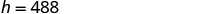The vertex is $\left(5.5,488\right)$ . Since the parabola has a maximum, the h- coordinate of the vertex is the maximum y -value of the quadratic equation. The maximum value of the quadratic is 488 feet and it occurs when $t=5.5$ seconds.

Aziza is solving this equation-2(1+x)=4x+10
No. 3^32 -1 has exactly two divisors greater than 75 and less than 85 what is their product?
x^2+7x-19=0 has Two solutions A and B give your answer to 3 decimal places
3. When Jenna spent 10 minutes on the elliptical trainer and then did circuit training for20 minutes, her fitness app says she burned 278 calories. When she spent 20 minutes onthe elliptical trainer and 30 minutes circuit training she burned 473 calories. How manycalories does she burn for each minute on the elliptical trainer? How many calories doesshe burn for each minute of circuit training?
.473
Angelita
?
Angelita
John left his house in Irvine at 8:35 am to drive to a meeting in Los Angeles, 45 miles away. He arrived at the meeting at 9:50. At 3:30 pm, he left the meeting and drove home. He arrived home at 5:18.
p-2/3=5/6 how do I solve it with explanation pls
P=3/2
Vanarith
1/2p2-2/3p=5p/6
James
Cindy
4.5
Ruth
is y=7/5 a solution of 5y+3=10y-4
yes
James
Cindy
Lucinda has a pocketful of dimes and quarters with a value of $6.20. The number of dimes is 18 more than 3 times the number of quarters. How many dimes and how many quarters does Lucinda have? Rhonda Reply Find an equation for the line that passes through the point P ( 0 , − 4 ) and has a slope 8/9 . Gabriel Reply is that a negative 4 or positive 4? Felix y = mx + b Felix if negative -4, then -4=8/9(0) + b Felix -4=b Felix if positive 4, then 4=b Felix then plug in y=8/9x - 4 or y=8/9x+4 Felix Macario is making 12 pounds of nut mixture with macadamia nuts and almonds. macadamia nuts cost$9 per pound and almonds cost $5.25 per pound. how many pounds of macadamia nuts and how many pounds of almonds should macario use for the mixture to cost$6.50 per pound to make?
Nga and Lauren bought a chest at a flea market for $50. They re-finished it and then added a 350 % mark - up Makaila Reply$1750
Cindy
the sum of two Numbers is 19 and their difference is 15
2, 17
Jose
interesting
saw
4,2
Cindy
Felecia left her home to visit her daughter, driving 45mph. Her husband waited for the dog sitter to arrive and left home 20 minutes, or 13 hour later. He drove 55mph to catch up to Felecia. How long before he reaches her?
hola saben como aser un valor de la expresión
NAILEA
integer greater than 2 and less than 12
2 < x < 12
Felix
I'm guessing you are doing inequalities...
Felix
Actually, translating words into algebraic expressions / equations...
Felix
hi
Darianna
hello
Mister
Eric here
Eric
6
Cindy

#### Get Jobilize Job Search Mobile App in your pocket Now!ByBy OpenStaxBy Qqq QqqBy Rohini AjayBy Nicole DuquetteBy Stephen VoronBy OpenStaxBy Madison ChristianBy OpenStaxBy Jams Kalo